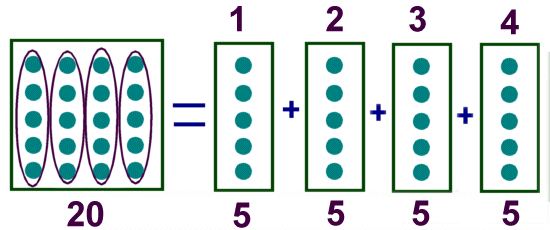# Kids Math

## Division Basics

What is division?

Division is breaking a number up into an equal number of parts.

Example:

20 divided by 4 = ?

If you take 20 things and put them into four equal sized groups, there will be 5 things in each group. The answer is 5.20 divided by 4 = 5.

Signs for Division

There are a number of signs that people may use to indicate division. The most common one is ÷, but the backslash / is also used. Sometimes people will write one number on top of another with a line between them. This is also called a fraction.

Example signs for "a divided by b":

a ÷ b
a/b
a
b

Dividend, Divisor, and Quotient

Each part of a division equation has a name. The three main names are the dividend, the divisor, and the quotient.
• Dividend - The dividend is the number you are dividing up
• Divisor - The divisor is the number you are dividing by
• Quotient - The quotient is the answer
Dividend ÷ Divisor = Quotient

Example:

In the problem 20 ÷ 4 = 5

Dividend = 20
Divisor = 4
Quotient = 5

Special Cases

There are three special cases to consider when dividing.

1) Dividing by 1: When dividing something by 1, the answer is the original number. In other words, if the divisor is 1 then the quotient equals the dividend.

Examples:

20 ÷ 1 = 20
14.7 ÷ 1 = 14.7

2) Dividing by 0: You cannot divide a number by 0. The answer to this question is undefined.

3) Dividend equals Divisor: If the dividend and the divisor are the same number (and not 0), then the answer is always 1.

Examples:

20 ÷ 20 = 1
14.7 ÷ 14.7 = 1

Remainder

If the answer to a division problem is not a whole number, the "leftovers" are called the remainder.

For example, if you were to try and divide 20 by 3 you would discover that 3 does not divide evenly into 20. The closest numbers to 20 that 3 can divide into are 18 and 21. You pick the closest number that 3 divides into that is smaller than 20. That is 18.

18 divided by 3 = 6, but there are still some leftovers. 20 -18 = 2. There are 2 remaining.

We write the remainder after an "r" in the answer.

20 ÷ 3 = 6 r 2

Examples:

12 ÷ 5 = 2 r 2
23 ÷ 4 = 5 r 3
18 ÷ 7 = 2 r 4

Division is the Opposite of Multiplication

Another way to think of division is as the opposite of multiplication. Taking the first example on this page:

20 ÷ 4 = 5

You can do the reverse, replacing the = with a x sign and the ÷ with an equal sign:

5 x 4 = 20

Examples:

12 ÷ 4 = 3
3 x 4 = 12

21 ÷ 3 = 7
7 x 3 = 21

Using multiplication is a great way to check your division work and get better scores on your math tests!

Back to Kids Math

Back to Kids Study